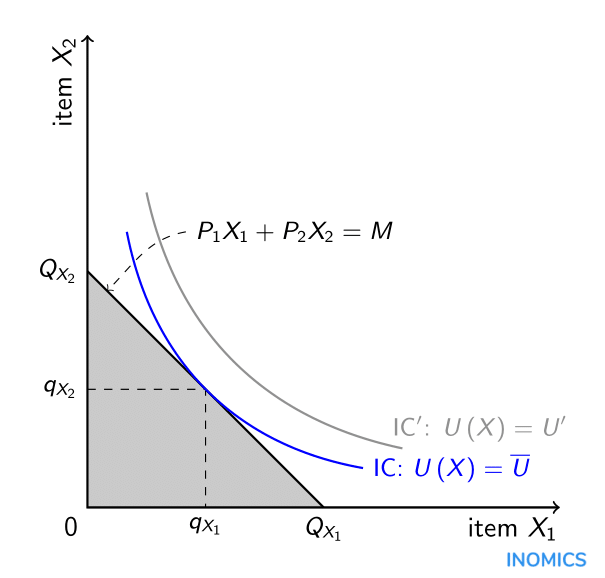# Utility Maximisation

Individuals derive utility from the consumption of goods and services, and choose these so as to maximise their utility under any given budget. The assumption that individuals maximise utility is a cornerstone of microeconomics. It is used as a basis to explain market behaviour and to predict how people act and react in particular situations. Implicitly, individuals are assumed to be perfectly rational, capable of calculating how much utility they will obtain from any combination of goods and services, and choosing the (or a) combination where utility hits a maximum, subject to a budget constraint.

In most economic modelling, an individual’s utility is described as a continuous, monotonous and concave function of consumption. That is, utility function U(X), where U stands for utility and X for consumption (X can be a vector of goods and services consumed), is continuous over the space of X. Its first derivative with respect to X is positive (“more is better”) and its second derivative with respect to X negative, representing diminishing marginal utility. The individual will, in most cases, be limited in consumption by a budget of income such that spending on goods and services X cannot exceed a maximum level M. The individual’s maximisation problem can be posed as

\begin{gather*} \max_{X}U\left(X\right)\\ \text{s.t.: }XP\leq M \end{gather*}

where P is the corresponding vector of prices for the goods and services X.

The graph depicts a simple two-dimensional case where the individual chooses between combinations of items X1 and X2 (item X2 might also represent “all other items” in order to simplify the presentation of the individual’s choice over X1, considering all available alternatives). Given the items’ prices P1 and P2 and the budget M, the individual could devote the entire budget to X1, purchasing QX1 at a cost of P1QX1 = M, or to X2, purchasing QX2 at a cost of P2QX2 = M. All affordable combinations of X1 and X2 are found in the grey shaded triangle which is limited by the budget line P1X1 + P2X2 = M running between the points (QX1,0) and (0,QX2). A set of indifference curves, each defined as combinations of X1 and X2 that give the individual a fixed level of utility (hence the indifference of the individual between such combinations), is drawn in the (X1,X2) space. Due to utility increasing in X, indifference curves further from the origin (0,0) represent higher levels of utility. The individual’s utility is hence maximised at the point where the budget line is tangential to the “highest” indifference curve IC at the point (qX1,qX2), resulting in utility Ū. On the indifference curve IC’ the individual would obtain even higher utility U’, however this is not attainable given the individual’s current budget M.Non-economists may balk at the idea that individuals calculate budget lines and indifference curves in order to make their consumption decisions. However this need not be the case for the theory still to have traction. It is sufficient for the analyst if individuals act as though they were maximising utility. Utility maximisation need not be a conscious or explicit process. What is important for an economist is to be able to observe behaviour and then explain what is observed. Decisions that are consistent with utility maximisation allow individual preferences to be summarised through utility functions, which is convenient for the analyst. Economists tend to value observing what people do (“revealed preference”) more highly than simply recording what they say they do or will do (“claimed preference”).

Insofar as the future state of the world is uncertain and decisions are made under such uncertainty, individuals can be assumed to maximise expected utility, which is the utility to be gained from each possible state of the world, weighted by the probabilities of the various states of the world being realised. A utility function can also be used to describe the individual’s attitude towards risk. For example, a risk-averse individual will tend to prefer lower but certain levels of consumption over potentially high but very uncertain levels of consumption. In this case the utility function will be strictly concave.